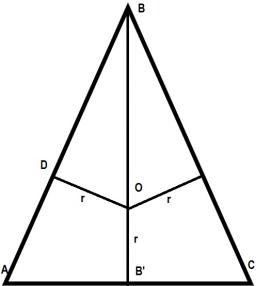# Circumference 4127

The base is 3.5 cm shorter than the shoulder in an isosceles triangle. The circumference of the triangle is 58 cm. Calculate the lengths of the sides of this triangle.

a =  20.5
b =  20.5
c =  17

### Step-by-step explanation:

a+b+c=58
a=b
c = a - 3.5

a+b+c = 58
a-b = 0
a-c = 3.5

a = 41/2 = 20.5
b = 41/2 = 20.5
c = 17

Our linear equations calculator calculates it.Did you find an error or inaccuracy? Feel free to write us. Thank you!

Tips for related online calculators
Do you have a linear equation or system of equations and looking for its solution? Or do you have a quadratic equation?